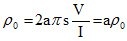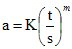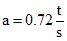Tel: (480) 219-9007

# Four Point Probe Equations

Courtesy of: ECE444: Theory and Fabrication of Integrated Circuits Electrical and Computer Engineering University of Illinois – Urbana/Champaign:

The four point probe, as depicted schematically in Figure 1, contains four thin collinear tungsten wires which are made to contact the sample under test. Current I is made to flow between the outer probes, and voltage V is measured between the two inner probes, ideally without drawing any current. If the sample is of semi-infinite volume and if the interprobe spacings are s1= s2 = s3 = s, then it can be shown that the resistivity of the semi-infinite volume is given byFigure 1

The subscript 0 in the preceding equation indicates the measured value of the resistivity and is equal to the actual value, ρ, only if the sample is of semi-infinite volume. Practical samples, of course, are of finite size. Hence, in general, ρ is not equal to ρ0. Correction factors for six different boundary configurations have been derived by Valdes1. These show that in general if l, the distance from any probe to the nearest boundary, is at least 5s, no correction is required. For the cases when the sample thickness is <= 5s, we can compute the true resistivity fromwhere a is the thickness correction factor which is plotted on page GT-2. From an examination of the plot we see that for values of t/s >= 5 the corresponding value of a is unity. Thus for samples whose thickness is at least 5 times the probe spacing, no correction factor is needed. Typical probe spacings are 25-60 mils and the wafers used in most cases are only 10-20 mils thick, so unfortunately we cannot ignore the correction factor. Looking again at the plot, however, we see that the curve is a straight line for values of t/s <= 0.5. Since it is a log-log plot the equation for the line must be of the formwhere K is the value of a at (t/s) = 1, and m is the slope. Inspection of the plot shows that in this case m = 1. K is determined to be 0.72 by extrapolating the linear region up to the value at (t/s) – 1. (The exact value can be shown to be 1/(2ln2).) Hence for slices equal to or less than one half the probe spacingWhen substituted into the basic equation we get:for t/s <= 0.5

All samples used in the lab satisfy the one-half relationship so the above formula can be used to determine ρ. Resistivity measurements will be performed on the starting material for each experiment. The value of ρ obtained will be referred to as the bulk resistivity, and the units are Ω-cm.

If both sides of Equation (4) are divided by t we getfor t/s <= 0.5 (5)

which we refer to as sheet resistance. When the thickness t is very small, as would be the case for a diffused layer, this is the preferred measurement quantity. Note that Rs is independent of any geometrical dimension and is therefore a function of the material alone. The significance of the sheet resistance can be more easily seen if we refer to the end-to-end resistance of a rectangular sample. From the familiar resistance formula(6)

we see that if w = l (a square) we getTherefore, Rs may be interpreted as the resistance of a square sample, and for this reason the units of Rs are taken to be Ohms per square or Ω/sq. Dimensionally this is the same as Ω, but this notation serves as a convenient reminder of the geometrical significance of sheet resistance.

So far in our discussion of resistivity measurements we have assumed that the size of our sample is large compared to the probe spacing so that edge effects could be ignored. This is usually the case for the bulk resistivity measurement. However, the sheet resistance measurements made in lab will be made on a “test area” of the wafer. The test area dimensions (nominally 2.9 by 5.8mm) are not that large compared to the probe spacing (25 mils). In order to get accurate measurements a correction factor for edge (geometry) effects must be included. The figure on page GT-3 gives the correction factors for two common sample geometries.

In general thenwhere C is the correction factor.

Note that for d/s > 40, C = 4.53, the value we had as the multiplier in Equation (5).

References

1. Valdes, L. G., Proc. I.R.E., 42, pp. 420-427 (February 1954)
2. Smits, F. M., “Measurements of Sheet Resistivity with the Four-Point Probe,” BSTJ, 37, p. 711 (1958). (Same as BT Monograph, 3894, Part 2)

Four-Point-Probes is a division of Bridge Technology. To request further information please call Bridge Technology at (480) 219-9007 or send e-mail to Joshua Bridge at: sales@bridgetec.com